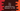# C++ program to find palindrome numbers between 1 to 100## Introduction :

I have discussed before on how to find all palindrome numbers in C++ in this post. If the reverse of a number is equal to the number itself, it is called a palindrome number.

The easiest way to check if a number is palindrome or not is by reversing the number. We can reverse the number and compare it with the original number to check if it is equal or not. If both are equal, it is a palindrome.

In the above post, I have explained two different ways to do that. One is by reversing the number itself and another one is by converting the number to a string and comparing each character from the start to middle with each character from end to middle. If all matches, it is not a palindrome. In both cases, we are comparing the first digit with the last digit of the number upto the mid position.

In this post, we will find all the palindrome numbers starting from 1 to 100. For that we will use one for loop that will check each number from 1 to 100 if it is palindrome or not. If a number is palindrome, it will print that out.

We will use the second approach that we have explained in the previous article i.e. we will convert the number to string and compare each first half digits with the second half digits. We will use one different method to do the checking. We are using for loop here but you can also use any other loop.

### Example program :

Let me show you the example before explaining in details :

``````using namespace std;
#include <iostream>
#include <cstring>

bool isPalindrome(int number)
{
// 3
string str_number = to_string(number);

// 4
int j = strlen(str_number.c_str()) - 1;

// 5
for (int i = 0; i < j; i++, j--)
{
if (str_number[i] != str_number[j])
{
// 6
return false;
}
}

return true;
}

int main()
{
// 1
for(int i =1; i<= 100; i++){
// 2
if(isPalindrome(i)){
cout<<i<<endl;
}
}
return 0;
}``````

### Explanation :

The commented numbers in the above program denote the step numbers below :

1. The main function is called first. Here, we are using one for loop to find out all palindrome numbers between 1 to 100. This loop runs from i = 1 to i = 100.
2. Inside the loop, we are calling isPalindrome method to check if the current value of i is palindrome or not. This method takes one integer number and returns one boolean value based on if the number is palindrome or not. If it returns true i.e. if the number is a palindrome, we are printing it to the user.
3. We are first converting the number to a string strnumber_ first.
4. We are finding out the length of the string i.e. total number of characters that this string has.
5. Using one for loop we are comparing all characters from the start to the middle with characters from end to the middle. i is used for forward iteration and j is used for backward iteration.
6. If any two characters are not equal, i.e. two digits of the number from left and right side are not equal, return false. If the for loop ends, return true i.e. it is a palindrome number.

### Output of the program :

This program will print the below output :

``````1
2
3
4
5
6
7
8
9
11
22
33
44
55
66
77
88
99``````

You can change the limit of the for loop to find palindrome numbers in any different range.